﻿ 基于最短路径的外卖配送时间优化问题研究

# 基于最短路径的外卖配送时间优化问题研究Research on Optimization of Takeaway Delivery Time Based on Shortest Path

Abstract: Aiming at the average waiting time of customers in the food delivery industry, a corresponding mathematical model is established based on the shortest path principle. Taking the order situation of customers in a certain area as the research object, using graph theory to abstract the geographic diagram of the area as an undirected weighted graph, building a customer waiting time model, and using Dijkstra algorithm to find the shortest distance between the two areas, using a random simulation algorithm to generate dynamic orders, and estimate the average waiting time of customers. On this basis, by changing the location of the distribution center and increasing the number of distribution personnel to shorten the average waiting time of customers, the effect of model optimization is achieved.

1. 引言

2. 顾客平均等待时间模型建立

2.1. 问题描述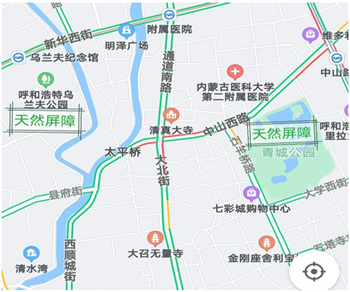Figure 1. Map around Inner Mongolia University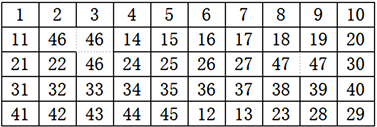Figure 2. Geographical sketch around Inner Mongolia UniversityTable 1. Customer order status table

2.2. 模型的准备工作

2.2.1. Dijkstra算法

Dijkstra算法是从一个顶点 ${u}_{0}$ 到G的其余各顶点的最短路径算法，用来解决有权图中最短路径问题。特点是从起点开始，每次遍历到距始点最近且未访问过的顶点的邻点，直到扩展到终点 ${v}_{0}$ 为止。具体步骤如下：

Step1：令 $I\left({u}_{0}\right)=0$，对 $v\ne {u}_{0}$，令 $I\left(v\right)=\infty$${S}_{0}=\left\{{u}_{0}\right\}$$i=0$

Step2：对每一个 $v\in \stackrel{¯}{{S}_{i}}\left(\stackrel{¯}{{S}_{i}}=V\{S}_{i}\right)$，用 $\underset{u\in {S}_{i}}{\mathrm{min}}\left\{I\left(v\right),I\left(u\right)+w\left(uv\right)\right\}$ 代替 $I\left(v\right)$，这里 $w\left(uv\right)$ 表示顶点u和v之间边的权值。计算 $\underset{u\in {S}_{i}}{\mathrm{min}}\left\{I\left(v\right)\right\}$，把达到这个最小的一个顶点记为 ${u}_{i+1}$，令 ${S}_{i+1}={S}_{i}\cup \left\{{u}_{i+1}\right\}$

Step3：若 $i=|V|-1$，则停止；若 $i<|V|-1$，则用 $i+1$ 代替i，转step2。

2.2.2. 最短送餐路径模型

${A}_{50×50}=\left[\begin{array}{ccccc}{s}_{1,1}& {s}_{1,2}& \cdots & {s}_{1,49}& {s}_{1,50}\\ {s}_{2,1}& {s}_{2,2}& \cdots & {s}_{2,49}& {s}_{2,50}\\ ⋮& ⋮& \ddots & ⋮& ⋮\\ {s}_{49,1}& {s}_{49,2}& \cdots & {s}_{49,49}& {s}_{49,50}\\ {s}_{50,1}& {s}_{50,2}& \cdots & {s}_{50,49}& {s}_{50,50}\end{array}\right]$

${s}_{i,j}=\left\{\begin{array}{l}1,\text{\hspace{0.17em}}\text{\hspace{0.17em}}当{v}_{i}和{v}_{j}之间有公路连通,\text{\hspace{0.17em}}\text{\hspace{0.17em}}i\ne j\\ \infty ,\text{\hspace{0.17em}}\text{\hspace{0.17em}}当{v}_{i}和{v}_{j}不相邻或由于46,47区阻隔时,\text{\hspace{0.17em}}\text{\hspace{0.17em}}i\ne j\\ 0,\text{\hspace{0.17em}}\text{\hspace{0.17em}}i=j\end{array}$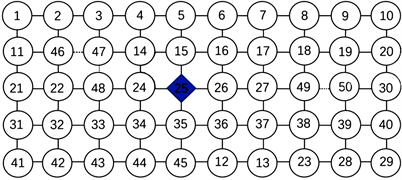Figure 3. Undirected weighted graph

2.3. 模型构建

1) 假设送餐员在公路上的平均行驶速度为30公里每小时。

2) 假设每块区域的所有顾客位置、餐馆位置和公司总部都位于该地区区域的左下角。

3) 假设商家和顾客服务时间之和服从正态分布 $N\left(\mu ,{\sigma }^{2}\right)$。其平均值是3分钟，方差为1分钟，即 $\mu =3,{\sigma }^{2}=1$

4) 忽略送餐员拐弯的时间，仅考虑沿道路行驶的时间。

${t}_{j}$ 为顾客的等待时间， ${t}_{hc}^{\left(j\right)}={T}_{\mathrm{min}}^{\left(j\right)}-{T}^{\left(j\right)}$ 为回程等待时间，即最快回到公司总部的送餐人到达公司的时

${T}_{\mathrm{min}}^{\left(j\right)}$ 与顾客下单时刻 ${T}^{\left(j\right)}$ 之差；其中 ${T}^{\left(j\right)}$ 为顾客下单的时间点， ${T}_{\mathrm{min}}^{\left(j\right)}=\mathrm{min}\left\{{T}_{s1}^{j},{T}_{s2}^{j},{T}_{s3}^{j},{T}_{s4}^{j},{T}_{s5}^{j},{T}_{s6}^{j}\right\}$ 为最先返回公司的送餐员到达公司的时间点。

3. 最短送餐路径模型和顾客等待时间模型求解

3.1. 算法概述

3.2. 算法步骤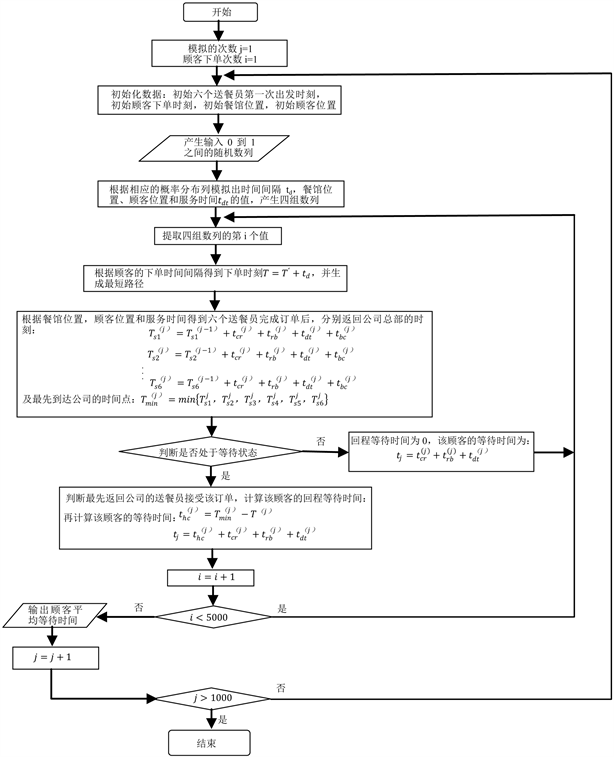Figure 4. Overall flow chart of the algorithm

Step1：用计算机产生n个[0,1]之间的随机数 ${\xi }_{\text{1}},{\xi }_{\text{2}},\cdots ,{\xi }_{n}$

Step2：令r = 1，判断 ${\xi }_{r}$，若 ${F}_{1}\left(k-1\right)\le {\xi }_{r}<{F}_{1}\left(k\right)$，( $k=1,2,\cdots ,36$ )，则使 ${\xi }_{r}=k$

Step3：判断r是否小于n，若是，令r = r + 1，返回Step2；否则，结束。

Step1：用计算机产生n个[0,1]之间的随机数 ${\xi }_{\text{1}},{\xi }_{\text{2}},\cdots ,{\xi }_{n}$

Step2：令r = 1，判断 ${\xi }_{r}$，若 ${\xi }_{r}={F}_{3}\left(k\right),\left(k\ge 0\right)$，则使 ${\xi }_{r}=k$

Step3：判断r是否小于n，若是，令r = r + 1，返回Step2；否则，结束。

3.3. 结果分析Table 3. Simulation solution results table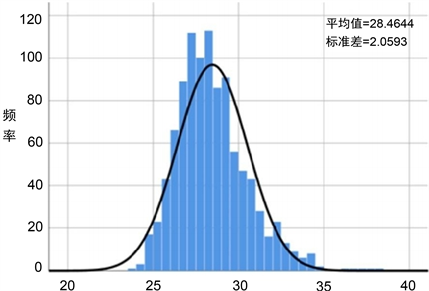Figure 5. Data distribution of simulation solution results

4. 基于配送中心选址和增加配送人员子方案的顾客平均等待时间优化

4.1. 基于配送中心选址子方案的顾客平均等待时间优化

4.2. 基于增加配送人员子方案的顾客平均等待时间优化

5. 结论

1) 该模型基于最短路径原则，大大缩短了配送距离和顾客平均等待时间，从而提高了配送效率。所以送餐人员可以在短时间内完成配送，提高顾客满意度，有助于外卖行业的长久发展。

2) 本文建立的模型可用于确定配送中心的位置以及最优配送人员数，且可为外卖配送优化问题提供较为新颖的求解思路，使外卖配送规划更具科学性，对外卖配送领域的研究有一定的贡献。

NOTES

*通讯作者。

 吴丽敏. 基于顾客满意度的多目标配送中心选址方法研究[J]. 物流科技, 2014(6): 95-97.

 杨粟涵, 于蕾. 基于遗传算法的快递配送路径优化问题研究[J]. 现代信息科技, 2020, 4(9): 99-103.

 范立南, 吕鹏. 基于改进遗传算法的校园外卖配送路径规划[J]. 物流科技, 2021(1): 14-19.

 黄驰, 黄耿石, 朱小玲. 基于遗传算法的送外卖最短路径研究[J]. 科技传播, 2016, 12(6): 94-95.

 翟劲松, 台玉红. 基于时间窗约束下的外卖配送路径优化[J]. 物流科技, 2018(3): 15-18.

Top